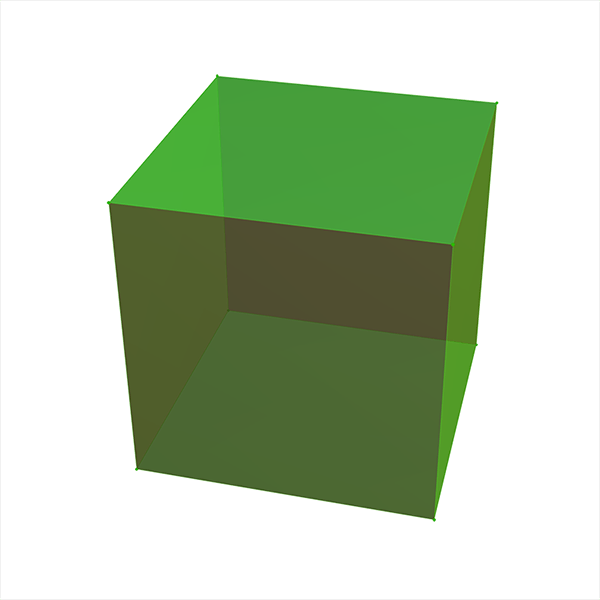## Glosarium

Pilih salah satu kata kunci di sebelah kiri…

# Graphs and NetworksPlanar Graphs

Waktunya membaca: ~25 min

Here is another puzzle that is related to graph theory.

In a small village there are three houses and three utility plants that produce water, electricity and gas. We have to connect each of the houses to each of the utility plants, but due to the layout of the village, the different pipes and cables are not allowed to cross.

Try to connect each of the houses to each of the utility companies below, without any of your lines intersecting:

Just like the Königsberg bridges before, you quickly discover that this problem is also impossible. It seems that some graphs can be drawn without overlapping edges – these are called planar graphs – but others cannot.

K3 is planar.

K4 .

K5 .

The complete graph K5 is the smallest graph that is not planar. Any other graph that contains K5 as a subgraph in some way is also not planar. This includes K6, K7, and all larger complete graphs.

The graph in the three utilities puzzle is the bipartite graph K3,3. It turns out that any non-planar graph must either contain a K5 or a K3,3 (or a subdivision of these two graphs) as a subgraph. This is called Kuratowski’s theorem.

### Planarity

This is a planar graph, but the \${n} vertices have been scrambled up. Rearrange the vertices so that none of the edges overlap.

## Euler’s Formula

All planar graphs divide the plane they are drawn on into a number of areas, called faces.

Vertices
Faces
Edges
11 Vertices + Faces

Vertices
Faces
Edges
15 Vertices + Faces

Vertices
Faces
Edges
25 Vertices + Faces

When comparing these numbers, you will notice that the number of edges is always than the number of faces plus the number of vertices. In other words, F + V = E + 1. This result is called Euler’s equation and is named after the same mathematician who solved the Königsberg Bridges problem.

Unfortunately, there are infinitely many graphs, and we can’t check every one to see if Euler’s equation works. Instead, we can try to find a simple proof that works for any graph…

 F V E 0 1 0

0 + 1  =  0 + 1

The simplest graph consists of a single vertex. We can easily check that Euler’s equation works.

Let us add a new vertex to our graph. We also have to add an edge, and Euler’s equation still works.

If we want to add a third vertex to the graph we have two possibilities. We could create a small triangle: this adds one vertex, one face and two edges, so Euler’s equation still works.

Instead we could simply extend the line by one: this adds one vertex and one edge, and Euler’s equation works.

Let’s keep going: if we now create a quadrilateral we add one vertex, two edges and one face. Euler’s equation still works.

Any (finite) graph can be constructed by starting with one vertex and adding more vertices one by one. We have shown that, whichever way we add new vertices, Euler’s equation is valid. Therefore, it is valid for all graphs.

The process we have used is called mathematical induction. It is a very useful technique for proving results in infinitely many cases, simply by starting with the simplest case, and showing that the result holds at every step when constructing more complex cases.

Many planar graphs look very similar to the nets of polyhedra, three-dimensional shapes with polygonal faces. If we think of polyhedra as made of elastic bands, we can imagine stretching them out until they become flat, planar graphs:This means that we can use Euler’s formula not only for planar graphs but also for all polyhedra – with one small difference. When transforming the polyhedra into graphs, one of the faces disappears: the topmost face of the polyhedra becomes the “outside”; of the graphs.

In other words, if you count the number of edges, faces and vertices of any polyhedron, you will find that F + V = E + .

Icosahedron
20 Faces
12 Vertices
30 Edges

Rhombicosidodecahedron
62 Faces
60 Vertices
120 Edges

Truncated Icosahedron
32 Faces (12 black, 20 white)
60 Vertices
90 Edges

Archie